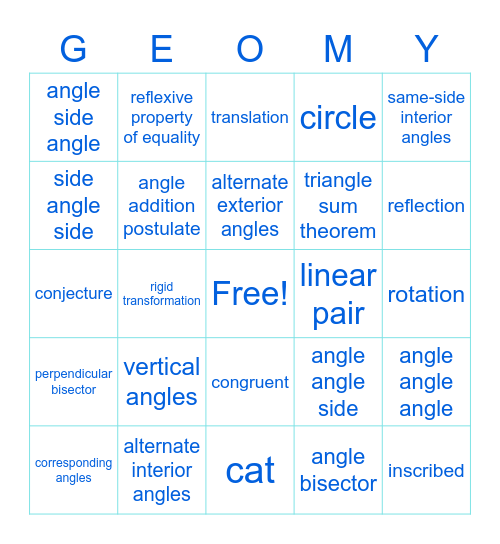# Angles and TrianglesThis bingo card has a free space and 24 words: vertical angles, alternate interior angles, alternate exterior angles, corresponding angles, same-side interior angles, angle side angle, side angle side, reflexive property of equality, linear pair, congruent, triangle sum theorem, conjecture, angle angle side, angle angle angle, angle addition postulate, angle bisector, rotation, translation, reflection, rigid transformation, inscribed, perpendicular bisector, circle and cat.

## Play Online